# Assessment of the Energy Distribution in Urban Areas by Using the Framework of Complex Network Theory

Assessment of the Energy Distribution in Urban Areas by Using the Framework of Complex Network Theory

Alberto Fichera Rosaria Volpe* Mattia Frasca

Dipartimento di Ingegneria Elettrica, Elettronica ed Informatica, viale Andrea Doria 6, 95125 Catania, Italy

Corresponding Author Email:
rvolpe@dii.unict.it
Page:
S430-S434
|
DOI:
https://doi.org/10.18280/ijht.34S234
|
Accepted:
|
Published:
31 October 2016
| Citation

OPEN ACCESS

Abstract:

The intense urbanization and the remarkable energy consumption deriving from urban areas are responsible for the 67% of the world energy demand. For that reason, the energy usage in cities has attracted considerable attention from governments and researchers. In this context, the diffusion of decentralized energy systems has progressively become more intense, causing the creation of energy interactions among consumers. In order to consider the energy exchanges deriving from those interactions, a new procedure based on the complex network theory has been developed. The elaborated mathematical tool consists of sorting the urban area into connected territorial units characterized by an energy demand and a potential energy generation. The procedure is tested for a speculative territory in order to determine the optimal energy flows occurring in the obtained urban energy network. Thus, the constructed model amounts to be a useful tool for the definition of urban energy action plans centered on the exploitation of renewable sources and on the installation of energy efficient systems.

Keywords:

City, Complex networks, Decentralized energy systems, Renewables.

1. Introduction

Energy consumption in cities is a central issue for urban planners, being cities responsible for the 67% of the world energy demand . The intense urbanization worldwide pushes towards the question of how to use the energy sources in a sustainable way reducing the dependence on fossil fuels and the consequential global warming. In this context, the diffusion of Decentralized Energy Systems (DES) has progressively become more intense due to the fact that DES allow to cover a significant share of the energy demand.

To the purpose, city governments have promoted several action plans for the integration of decentralized energy systems in their territories [2, 3, 4]. For the case of Italy, an energy policy initiative focuses on the possibility to generate and distribute energy locally . The energy usage is a topic also treated by researchers. Webb et al.  provide an empirical overview and analysis of the UK local government sustainable energy plans leading to sustainable decentralized energy systems. In the work of Chmielewski et al.  attention has been directed towards the diffusion of micro-cogeneration systems in Poland.

Regarding the optimization of decentralized energy systems, many studies can be find in the literature. In this direction, the work of Villatoro Flores et al.  addresses the selection of generation technologies for a decentralized energy system based  on  local  renewable  sources.  Similarly, Chauhan and Saini  optimize a renewable energy system for rural areas in India. Katsoulakos and Kaliampakos  present a linear optimization model for the improvement of decentralized energy systems in mountainous areas. Semache et al.  study the impact of a solar system on the energy performances of the rural housing in Algeria.

Yet, the diffusion of DES at urban scale results on the creation of energy interactions among consumers, which can be identified as buildings, neighborhoods or municipalities. In this sense, consumers have the possibility to exchange their own produced energy. Therefore, the decentralization of the urban energy systems requires the definition of mathematical methods, as formerly developed by Fichera et al. , which take the energy exchange among consumers into account, and permit the optimization of the energy flows within the neighborhood. In this direction, the presented paper develops the concept of urban energy network on the ground of the complex network theory. The main advantage of complex networks models resides on the possibility to analyze the interactions that can occur among its constituents . In fact, the concepts of nodes and links, typically used for the study of networks , can be transferred to the energy context, by considering nodes as consumers and links as the interactions for the exchange of energy.

Therefore, in this paper a mathematical tool, which is based to optimize the energy flows occurring between autonomous consumers, is presented.

2. Model Description

The matching of energy demands and energy generation of consumers at a neighborhood scale is evaluated in this section by introducing a mathematical model based on the complex network theory.

Networks are constituted by nodes and links. In the context of an urban energy networks, nodes stand for consumers with a given energy demand and the potential to install renewable energy sources for the autonomous production of energy. In this sense, consumers use the generated energy primarily for the satisfaction of their own energy demand and, then, distribute the energy exceed to their neighbors. The links of the networks represent the connections for the exchange of energy.

More in detail, N nodes are distributed on the space. Each node i, for i=1,…,N, is characterized by an energy demand Di and a potential energy generation Gi. Links are established according to a neighborhood criterion, for which two nodes may exchange energy if their distance d is under a given threshold. This hypothesis derives from the guideline of the Italian Regulation . Besides the potential connections with the neighbors, each node has a further connection with the power station, hereinafter called central node.

All interactions are described in a (N+1) x (N+1) matrix, called the adjacency matrix A. The elements aij represent the connection between node i and node j, being i, j = 1, … ,N+1 with i j. If aij = 1 there is a link between node i and node j; otherwise, if aij = 0 no link exists between node i and node j.  No interactions can take place between a node and itself; this condition is expressed by setting zeros in the diagonal of the adjacency matrix A.

Once the topology of the energy connections among nodes is defined, the model takes into account the evaluation of the energy status of each node. In order to do this, a surplus parameter Si = Gi - Di is introduced.

Three main conditions can occur:

• a node has a positive energy surplus and is therefore able to distribute its energy exceed to the neighbors;
• a node has a negative energy surplus and needs to receive energy from its neighbors or eventually from the central node;
• a node has totally fulfilled its energy demands and has no energy exceed to distribute.

The sign of the surplus parameters specifies the direction of the energy flows within the urban energy network. In fact, all energy exchange may take place only from a node with a positive energy surplus to a node with a negative energy surplus. The elements if the adjacency matrix are updated according to the sign of the surpluses. More specifically, aij = 1 if the energy exchange takes place from node i and node j; aij = - 1 if the energy exchange follows the direction from node i and node j. Finally, aij = 0 if no exchange occurs. Regarding the central node, it has a nil energy demand and its energy generation is equal to the residual energy demand that is not covered from the energy distribution deriving from the production of the nodes.

The optimization problem can be formulated as finding the optimal energy flows among nodes by minimizing the energy output deriving from the central node.

In order to determine the energy flows Xijoccurring between two nodes i and j, the problem is formulated as a linear programming problem.  Each node of the network has to respect the following energy balance.

$S_{i}=\sum_{j=1}^{N+1} a_{i j} X_{i j}, \forall i=1, \ldots,(N+1), i \neq j$(1)

The objective function of the model minimizes all energy flows exiting from the central node, thus avoiding any energy exchange between the central node and a node with a positive surplus. It is expressed as follows.

$\min \sum_{a_{1 j>0}} a_{1 j} X_{1 j}$(2)

The constrain of the model impose that all energy flows are non-negative.

$X_{i j}>0$(3)

After the calculation of the energy flows exchanged between nodes, the study aims to evaluate the exploitation of the urban energy network for the energy distribution. For this purpose, the starting topology of the urban energy network, for which the connections between two nodes were established on the ground of a neighborhood criterion, is compared with the optimized topology characterized by the links effectively used for the energy exchanges.

In order to quantify this exploitation, a network index is introduced and is defined as follows.

$I_{N}=\frac{\operatorname{link} S_{e_{x p \text {loited}}}}{\operatorname{link} S_{\text {neighborhood}}}$(4)

The index IN varies within the interval 0 < IN < 1. Particularly, IN = 0 means that none of the links of the starting topology are used for the energy distribution. On the other side, IN = 1 correspond to the exploitation of all links. Obviously, the larger is IN, the more exploited are the connections among nodes.

3. Results and Discussion

The results of the application of the described methodology are given and discussed in this section.

Two main questions are followed; on one side to determine the optimal exploitation of the urban energy network by varying the energy generation and, on the other side to investigate if the distance of connection between two nodes influences the results.

For the present study, the urban energy network is applied on a speculative territory. The chosen territory consists of N=500 nodes distributed within an area of 1000 m2 and connected with the power station as shown in Figure 1. Nodes are represented as blue stars, the power station is arranged in the bottom left of the figure and its connections with each node are highlighted through green links.

Each node of the network has an energy demand Di and an energy generation Gi. The energy demand Di varies within the interval [2 MWh, 7 MWh] according to a random uniform distribution. The energy generation Gi is expressed as a percentage of the total energy demand of the network, i.e. $\sum_{i=1}^{N} D i$ .

## figure_1.jpg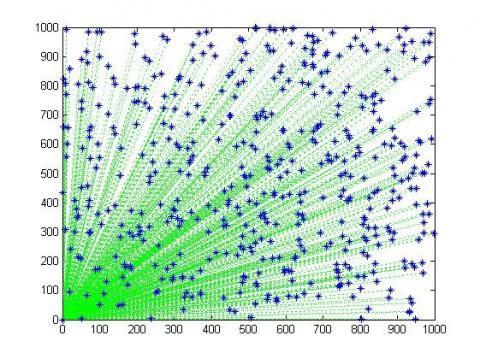Figure 1. Nodes of the chosen territory and their connections to the power station

Figure 2 shows the urban energy networks for different values of distance d of connection. By increasing the distance of connections, the number of links increase because of a more widespread neighborhood. In the figure, red links stand for the connections among nodes.

The urban energy networks in Figure 2 represents the starting point for the implementation of the model. As before stated, the aim of the optimization is to minimize the energy flows exiting from the central node. Once the urban energy network is solved, the model maintains only those links for which any energy exchange takes place.

## figure_2a.jpg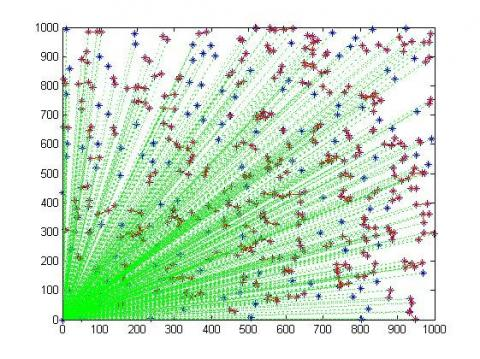(a)

## figure_2b.jpg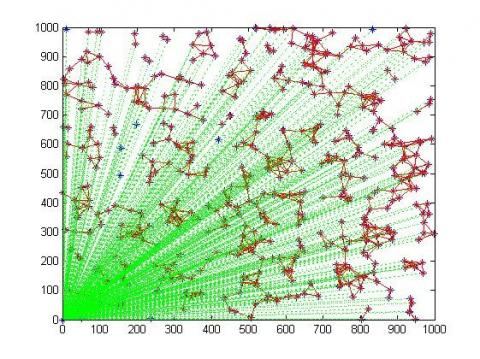(b)

## figure_2c.jpg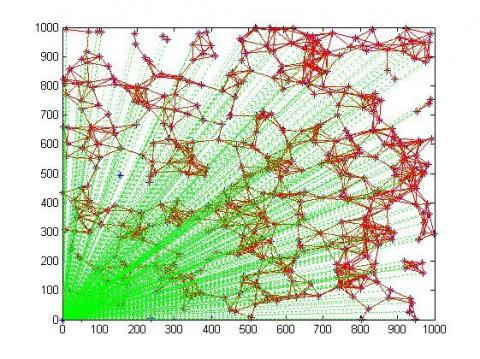(c)

Figure 2. (a) Urban energy network for a distance of 30 m; (b) Urban energy network for a distance of 50 m; (c) Urban energy network for a distance of 70 m

In order to evaluate if the number of links in the optimal urban energy networks differ from the number of links in the initial patterns of Figure 2, the network index IN is calculated. The results are shown in Figure 3.

## figure_3.png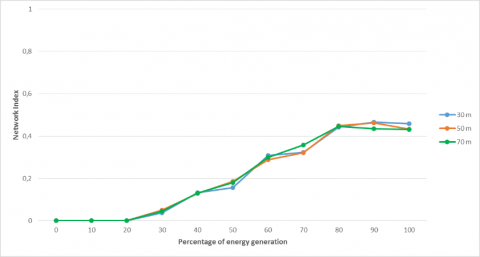Figure 3. Network index by varying the percentage of the energy generation at given distances

The results show that the network index increases linearly until the 80% of the energy generation, for which its value is approximately IN  = 0,46 for the three distances. This conditions means that the number of links effectively exploited for the exchange of energy is around the 46% of all feasible connections deriving from the neighborhood criterion. Beyond this value, the integration of renewable sources on the territory does not guarantee that the number of exploited connections increases. This is due to the fact that locally demands and generations mismatch and the supply of energy still depends from the central node.

Moreover, the results are not sensitive to the distance d. In fact, by varying the distance of connection among nodes, the number of exploited connections is proportionally the same.

As example, the optimal urban energy networks corresponding to the 80% of energy generation are exposed in Figure 4.

## figure_4a.jpg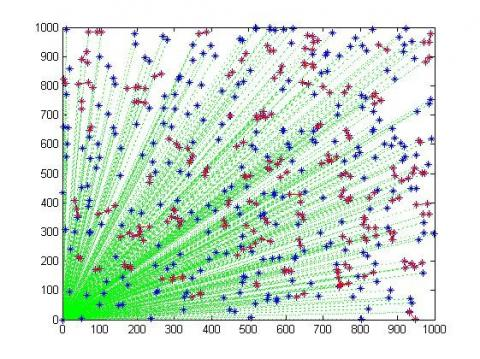(a)

## figure_4b.jpg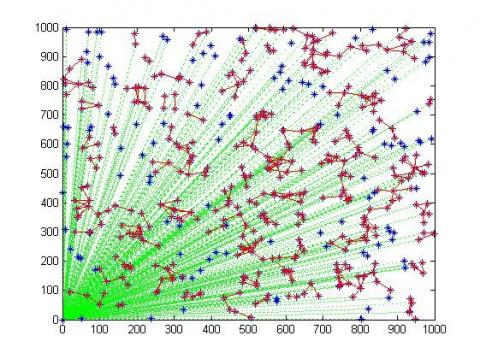(b)

## figure_4c.jpg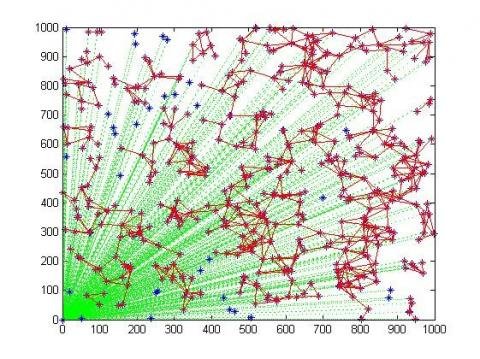(c)

Figure 4. (a) Optimized urban energy network for a distance of 30 m; (b) Optimized urban energy network for a distance of 50 m; (c) Optimized urban energy network for a distance of 70 m

As can be seen from Figure 4 and in comparison with Figure 2, the number of links decreases for each urban energy network. Instead, some of the connections with the power station are still required for the satisfaction of the energy demand of the network.

4. Conclusions

In this paper a new mathematical model based on the complex network theory and its application are introduced for the analysis of the energy distribution in urban areas. Consumers are characterized as nodes by a particular energy profile, i.e. with an energy demand and a potential energy generation. Nodes connect according to a spatial criterion and the established interactions are represented as links. The mathematical procedure is formulated as a linear programming problem and has the main objective to maximize the energy exchange occurring between autonomous consumers that have installed distributed energy systems.

The model has been tested for a hypothesized territory of 1000 m2 with a homogeneous distribution of consumers. In particular, the study has focuses on the determination of the optimal exploitation of the urban energy network by varying the energy generation and on the investigation of the influence of the distance d of connection between two nodes.

The results permit to conclude that at most only the 46% of all feasible links of the urban energy network are effectively exploited for the exchange of energy. This result occurs in correspondence of an amount of generation corresponding to the 80% of the total energy demand of the network.

In addition, the results are not sensitive to the distance of connection. Further consideration may include in the study a cost analysis of the energy distribution within the urban energy network.

Nomenclature
 N number of nodes Di energy demand of each node i [MWh] Gi energy generation of each node i [MWh] d distance of connection between two nodes of the network [m] A adjacency matrix of the network aij elements of the adjacency matrix Si energy surplus that characterize each node i Xij energy flow exchanged between node i and node j IN network index linksexploited number of links that are effectively exploited for the energy distribution on the urban energy network linksneighborhood number of links of the starting urban energy network and defined through the neighborhood criterion
References

 International Energy Agency, Key World Energy STATISTICS, Edition 2015, available at http://www.iea.org/publications/freepublications/publication/key-world-energy-statistics-2015.html

 A. M. Adil and Y. Ko, “Socio-technical evolution of Decentralized Energy Systems: A critical review and implications for urban planning and policy,” Renewable and Sustainable Energy Reviews, vol. 57, pp. 1025-1037, May 2016.  DOI: 10.1016/j.eser.2015.12.079.

 A. R. Neves, V. Leal and J. C. Lourenco, “A methodology for sustainable and inclusive local energy planning,” Sustainable Cities and Society, vol. 17, pp. 110-121, April 2015. DOI: 10.1016/j.scs.2015.04.005.

 C. Brandoni and F. Polonara, “The role of municipal energy planning in the regional energy-planning process,” Energy, vol. 48, pp. 323-338, July 2012. DOI: 10.1016/j.energy.2012.06.061.

 Delibera del 7 agosto 2014 426/2014/R/EEL, available at http://www.gse.it/it/Qualifiche%20e%20certificati/Qualifiche_SEU_SEESEU/Pagine/default.aspx (in Italian)

 J. Webb, D. Hawkey and M. Tingey, “Governing cities for sustainable energy: The UK case,” Cities, vol. 54, pp. 28-35, December 2016. DOI: 10.1016/j.cities.2015.10.014.

 A. Chmielewski, R. Guminski, J. Maczak, S. Radkowski and P. Szulim, “Aspects of balanced development of RES and distributed micro-cogeneration use in Poland: Case study of a μCHP with Stirling engine,” Renewable and Sustainable Energy Reviews, vol. 60, pp. 930-952, February 2016. DOI: 10.1016/j.rser.2016.01.131.

 H. F. Villatoro Flores, T. Furubayashi and T. Nakata, “Decentralized electricity generation system based on local renewable energy sources in the Honduran rural residential sector,” Clean Technologies and Environmental Policy, vol. 18, pp. 883-900, July 2015. DOI: 10.1007/s10098-015-1067-x.

 A. Chauhan and R. P. Saini, “Discrete harmony search base size optimization of Integrated Renewable Energy System for remote rural areas of Uttarakhand state in India,” Renewable Energy, vol. 94, pp. 587-604, April 2016. DOI: 10.1016/j.renene.2016.03.079.

 N. M. Katsoulakos and D. C. Kaliampakos, “Mountainous areas and decentralized energy planning: Insights from Greece,” Energy Policy, vol. 91, pp. 174-188, January 2016. DOI: 10.1016/j.enpol:2016.01.007.

 A. Semanche, A. Hamidat and A. Benchatti, “Impact study of the solar energy on the energy performances of the rural housing in Algeria,” International Journal of Heat and Technology, vol. 33 (2015). No. 4, pp. 229-236. DOI: 10.18280/ijht.330431.

 A. Fichera, L. Fortuna, M. Frasca and R. Volpe, “Integration of complex networks for urban energy mapping,” International Journal of Heat and Technology, vol. 33 (2015), No.4, pp. 181-184. DOI: 10.18280/ijht.330423.

 M. E. J. Newman, Networks: An introduction. Oxford, UK: Oxford University Press, 2010.